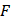# In the following question there are four terms.  The terms right to the symbol : : have same relationship as the two terms to the left of symbol : :.  Out of the four terms one term is missing, which is shown by (?) and which is one of the below.  Find out the correct alternative. BHC : FLG : : JPK : ? (a) MSP (b) NTO (c) EKF (d) SYT

## Question ID - 101275 :- In the following question there are four terms.  The terms right to the symbol : : have same relationship as the two terms to the left of symbol : :.  Out of the four terms one term is missing, which is shown by (?) and which is one of the below.  Find out the correct alternative. BHC : FLG : : JPK : ? (a) MSP (b) NTO (c) EKF (d) SYT

3537

NTO

Next Question :

A uniform rod of masskg and lengthis suspend by two smooth hinges 1 and 2 as shown in Fig. A forceN is applied downward at a distancefrom hinge 2. Due to the application of forcehinge 2 breaks. At this instant, applied forceis also removed. The rod starts to ratate downward about hinge 1.)The reaction at hinge 1, before hinge 2 breaks, is a) 24N b) 12N c) 11N d) 10N